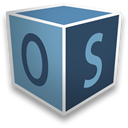OpenStudio:utilities
openstudio::Quantity Class Reference

#include <Quantity.hpp>

Public Member Functions

Constructors
Quantity (const UnitSystem &system=UnitSystem::Mixed)

Quantity (double value, const UnitSystem &system=UnitSystem::Mixed)

Quantity (double value, const Unit &units)

Quantity (const Quantity &q)

Quantityoperator= (const Quantity &q)

virtual ~Quantity ()

Value
double value () const

void setValue (double newValue)
Value setter. More...

Units
Unit units () const
Returns deep copy (clone) of this Quantity's units. More...

UnitSystem system () const

bool isTemperature () const
Returns true if units().optionalCast<TemperatureUnit>(). More...

std::vector< std::string > baseUnits () const
Vector of base units available to the Quantity. More...

int baseUnitExponent (const std::string &baseUnit) const
Base unit exponent getter. More...

void setBaseUnitExponent (const std::string &baseUnit, int exponent)
Sets the exponent of a base unit in this Quantity's m_units. More...

std::string standardUnitsString (bool withScale=true) const
Units in string form. More...

std::string prettyUnitsString (bool withScale=true) const
Pretty string for this quantity's units. More...

void setPrettyUnitsString (const std::string &str)
Sets the prettystring for this Quantity's m_units. More...

Scale scale () const
Scale getter. More...

bool setScale (int scaleExponent)
Sets the scale to the one registered in ScaleFactory for 10^scaleExponent. More...

bool setScale (const std::string &scaleAbbreviation)
Sets the scale to the one registered in ScaleFactory under scaleAbbreviation. More...

Temperature Methods

Quantities that have units of temperature need several extra functions to distinguish between absolute and relative measures.

bool isAbsolute () const
Throws if !units().optionalCast<TemperatureUnit>(). More...

bool isRelative () const
Throws if !units().optionalCast<TemperatureUnit>(). More...

void setAsAbsolute ()
Set temperature as absolute. More...

void setAsRelative ()
Set temperature as relative. More...

IP Methods
void lbmToLbf ()
Conversions for pounds mass to pounds force. More...

void lbfToLbm ()
Conversions for pounds mass to pounds force. More...

Mathematical Operators
Quantityoperator+= (const Quantity &rQuantity)

Quantityoperator-= (const Quantity &rQuantity)
Subtract and assign operator. More...

Quantityoperator*= (const Quantity &rQuantity)
Multiply and assign operator. More...

Quantityoperator/= (const Quantity &rQuantity)
Divide and assign operator. More...

Quantityoperator*= (double d)
Multiply by double. More...

Quantityoperator/= (double d)
Divide by double. More...

Quantitypow (int expNum, int expDenom=1)
Raise Quantity to a rational power. More...

Unit m_units

double m_value

Friends

std::ostream & operator<< (std::ostream &os, const Quantity &q)

Related Functions

(Note that these are not member functions.)

std::ostream & operator<< (std::ostream &os, const Quantity &q)

typedef boost::optional< QuantityOptionalQuantity

typedef std::vector< QuantityQuantityVector

Detailed Description

Quantity class defines value, unit pairs.

Units are set at construction and are modified by arithmetic operations. The constructors and assignment operator ensure that this class behaves as "plain old data" (POD) by cloning any units information (to avoid multiple objects pointing to the exact same unit data).

Constructor & Destructor Documentation

 openstudio::Quantity::Quantity ( const UnitSystem & system = UnitSystem::Mixed )
explicit
 openstudio::Quantity::Quantity ( double value, const UnitSystem & system = UnitSystem::Mixed )
explicit
 openstudio::Quantity::Quantity ( double value, const Unit & units )
 openstudio::Quantity::Quantity ( const Quantity & q )
 virtual openstudio::Quantity::~Quantity ( )
inlinevirtual

Member Function Documentation

 int openstudio::Quantity::baseUnitExponent ( const std::string & baseUnit ) const

Base unit exponent getter.

Returns 0 if baseUnit not present.

 std::vector openstudio::Quantity::baseUnits ( ) const

Vector of base units available to the Quantity.

 bool openstudio::Quantity::isAbsolute ( ) const

Throws if !units().optionalCast<TemperatureUnit>().

 bool openstudio::Quantity::isRelative ( ) const

Throws if !units().optionalCast<TemperatureUnit>().

 bool openstudio::Quantity::isTemperature ( ) const

Returns true if units().optionalCast<TemperatureUnit>().

 void openstudio::Quantity::lbfToLbm ( )

Conversions for pounds mass to pounds force.

These functions will throw if !units().optionalCast<IPUnit>().

 void openstudio::Quantity::lbmToLbf ( )

Conversions for pounds mass to pounds force.

These functions will throw if !units().optionalCast<IPUnit>().

 Quantity& openstudio::Quantity::operator*= ( const Quantity & rQuantity )

Multiply and assign operator.

Throws if Quantity is a particular system and rQuantity is not the same system.

 Quantity& openstudio::Quantity::operator*= ( double d )

Multiply by double.

No impact to units or scale. Does not throw.

 Quantity& openstudio::Quantity::operator+= ( const Quantity & rQuantity )

Throws if units are not equal.

 Quantity& openstudio::Quantity::operator-= ( const Quantity & rQuantity )

Subtract and assign operator.

Throws if units are not equal.

 Quantity& openstudio::Quantity::operator/= ( const Quantity & rQuantity )

Divide and assign operator.

Throws if Quantity is a particular system and rQuantity is not the same system.

 Quantity& openstudio::Quantity::operator/= ( double d )

Divide by double.

No impact to units or scale. Does not throw.

 Quantity& openstudio::Quantity::operator= ( const Quantity & q )
 Quantity& openstudio::Quantity::pow ( int expNum, int expDenom = 1 )

Raise Quantity to a rational power.

Throws openstudio::Exception if expDenom is not a common divisor for all baseUnit and scale exponents. Returned scale exponent may differ from expectation based on initialization of openstudio::ScaleFactory, see Scale operators declared in ScaleFactory.hpp.

 std::string openstudio::Quantity::prettyUnitsString ( bool withScale = true ) const

Pretty string for this quantity's units.

For instance N = kg*m/s^2.

Unit::prettyUnitsString
 Scale openstudio::Quantity::scale ( ) const

Scale getter.

 void openstudio::Quantity::setAsAbsolute ( )

Set temperature as absolute.

Throws if !units().optionalCast<TemperatureUnit>().

 void openstudio::Quantity::setAsRelative ( )

Set temperature as relative.

Throws if !units().optionalCast<TemperatureUnit>().

 void openstudio::Quantity::setBaseUnitExponent ( const std::string & baseUnit, int exponent )

Sets the exponent of a base unit in this Quantity's m_units.

This function will throw if baseUnit is not a valid option for this type of unit. A generic Unit allows any base unit to be set.

 void openstudio::Quantity::setPrettyUnitsString ( const std::string & str )

Sets the prettystring for this Quantity's m_units.

openstudio::Unit attempts to keep up with prettyStrings, but makes no promises. Precondition: isCompoundUnit(str). Otherwise throws.

 bool openstudio::Quantity::setScale ( int scaleExponent )

Sets the scale to the one registered in ScaleFactory for 10^scaleExponent.

 bool openstudio::Quantity::setScale ( const std::string & scaleAbbreviation )

Sets the scale to the one registered in ScaleFactory under scaleAbbreviation.

 void openstudio::Quantity::setValue ( double newValue )

Value setter.

 std::string openstudio::Quantity::standardUnitsString ( bool withScale = true ) const

Units in string form.

LaTeX mathematical formatting–_ for subscripting, ^ for superscripting. Curly braces {} are used for grouping.

Unit::standardUnitsString
 UnitSystem openstudio::Quantity::system ( ) const
 Unit openstudio::Quantity::units ( ) const

Returns deep copy (clone) of this Quantity's units.

 double openstudio::Quantity::value ( ) const

Friends And Related Function Documentation

 std::ostream& operator<< ( std::ostream & os, const Quantity & q )
friend
 std::ostream & operator<< ( std::ostream & os, const Quantity & q )
related
 typedef boost::optional OptionalQuantity
related
 typedef std::vector QuantityVector
related

Member Data Documentation

 Unit openstudio::Quantity::m_units
protected
 double openstudio::Quantity::m_value
protected